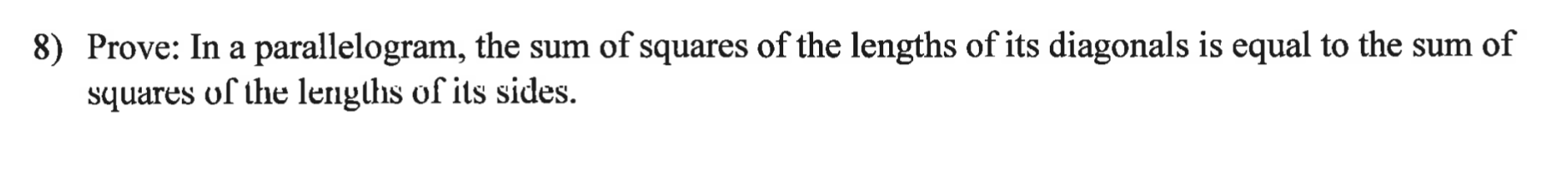# 8) Prove: In a parallelogram, the sum of squares of the lengths of its diagonals is equal to the sum of squares of the lengths of its sides.

Question

help!! the assignment is on quadrilaterals, similar triangles, and circles! I really need this answered! answer in paragraph proofhelp_outlineImage Transcriptionclose8) Prove: In a parallelogram, the sum of squares of the lengths of its diagonals is equal to the sum of squares of the lengths of its sides. fullscreen